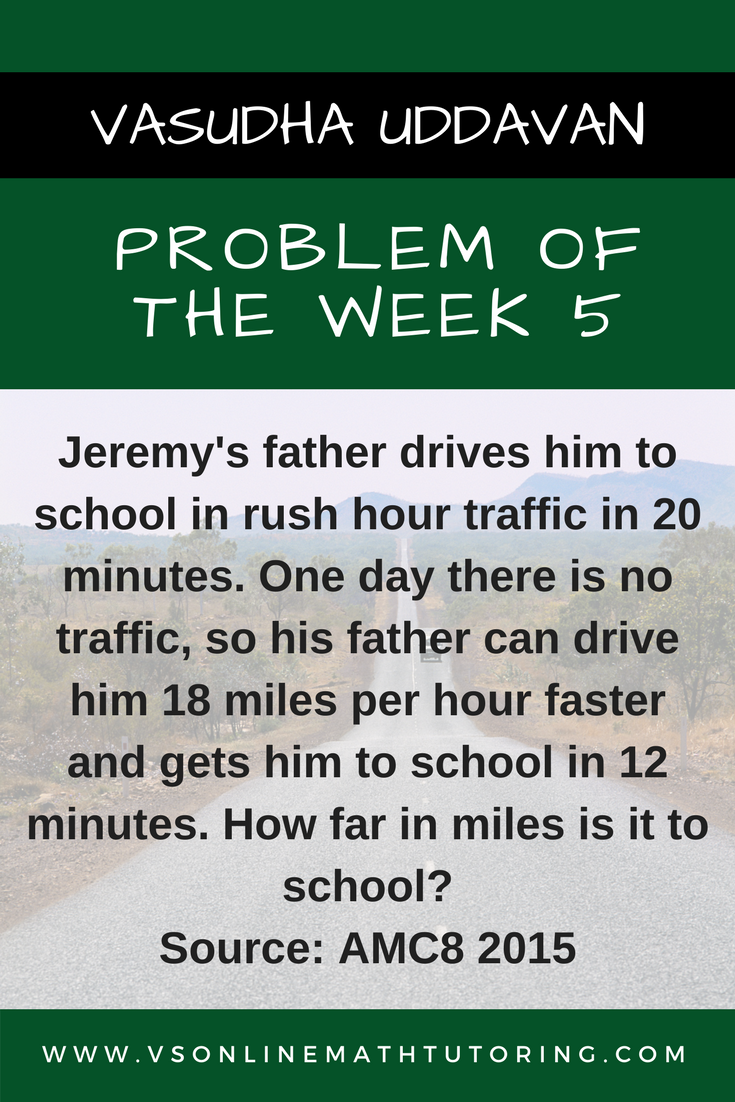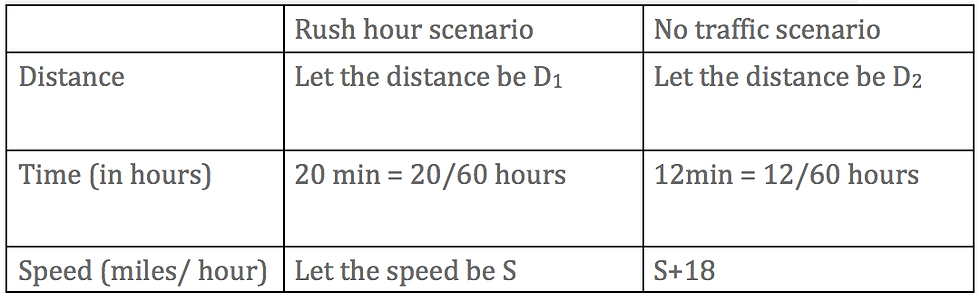top of pageSearch

# Math Competition Problems Problem of the Week - 5

Updated: Oct 18, 2020

##### Source: AMC8 2015Oh good. Another distance-time-speed question :) The one that always troubles all middle schoolers and probably some high-schoolers too!

So this question has 2 scenarios. One with traffic and another without. So let’s do a table for both scenarios. We need to see how they both relate to each other. That’ll help us solve the question so much faster.Distance = Speed * Time

D1 = S * 20/60

D2 = (S+18) * 12/60

Since D1 = D2 (They are travelling the same distance from home to school)

S * 20/60 = (S+18) * 12/60

Multiplying both sides by 60 we get

20S = 12S + 216

8S = 216

S = 27

Now that we found that S = 27 miles/ hr, let’s plug it back to the first equation

D1 = S * 20/60 to get our distance

D1 = 27 * 20/60

= 27 *1/3

= 9 miles

So it is 9 miles to school

Summary - if you get a distance-time-speed question with 2 scenarios, always make a table to see how the scenario variables relate to each other. It makes the problem so much more easy to solve

Now you try a similar problem

A cargo plane flew to the maintenance facility and back. It took one hour less time to get there than it did to get back. The average speed on the trip there was 220 mph. The average speed on the way back was 200 mph. How many hours did the trip there take?